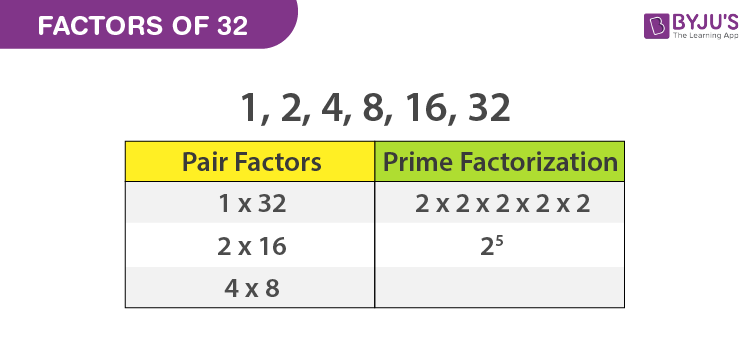Checkout JEE MAINS 2022 Question Paper Analysis : Checkout JEE MAINS 2022 Question Paper Analysis :

# Factors of 32

Factors of 32 are the integers that can divide 32, completely. If we say, x is a factor of 32, then 32 is evenly divisible by x. Since 32 is a composite number, therefore it will have more than two factors.

Factor pairs of the number 32 are the whole numbers which could be either positive or negative but not a fraction or decimal number. We can use the factorization method to find the factors of a number.## Pair Factors of 32

To find the factor pairs of 32, multiply the two numbers in a pair to get the original number as 32, such numbers are as follows:

 Positive Pair Factors Negative Pair Factors 1 × 32 = 32 ⇒ (1, 32) -1 × -32 = 32 ⇒ (-1, -32) 2 × 16 = 32 ⇒ (2, 16) -2 × -16 = 32 ⇒ (-2, -16) 4 × 8 = 32 ⇒ (4, 8) -4 × -8 = 32 ⇒ (-4, -8)

## How to calculate the Factors of 32?

To find the factors of 32 we need to divide the integer 32 by all the natural numbers from 1 to 32. The numbers that can divide 32 evenly or completely are the factors.

First of all, we know, any natural number is also a factor of itself.

32 ÷ 1 = 32

Now, we can see 32 is an even number, thus, it is divisible by 2 but not by any odd number (in this case). Therefore,

32 ÷ 2 = 16

Let us check with other even numbers.

32 ÷ 4 = 8

32 ÷ 8 = 4

32 ÷ 16 = 2

32 ÷ 32 = 1

Therefore, there are total six factors of the whole number 32.

 Factors of 32 1, 2, 4, 8, 16 and 32

## Prime Factors of 32

The number 32 is a composite and it should have prime factors. Now let us know how to calculate the prime factors of 32.

Step 1: The first step is to divide the number 32 with the smallest prime factor, say 2.

32 ÷ 2 = 16

Step 2: Again divide 16 by 2 and the process goes on.

16 ÷ 2 = 8

8 ÷ 2 = 4

4 ÷ 2 = 2

2 ÷ 2 = 1

Finally, we received the number 1 at the end of the division process. So that we cannot proceed further. So, the prime factors of 32 are written as 2 × 2 x 2 x 2 x 2 or 25, where 2 is a prime numbers.

It is possible to find the exact number of factors of a number with the help of prime factorisation. The prime factor of the 32 is 25. The exponent in the prime factorisation is 5. When you add the number 1 with the exponent, we get 6. i.e.,5 +1 = 6. Therefore, the number 32 has 6 factors.

## Video Lesson on Prime Factors## Solved Examples

Q.1: If there are 32 toffees to be distributed among 4 students in a class. How many toffees does each student get?

Solution: Given,

Number of toffees = 32

Number of students = 4

Each student will get = 32/4 = 8 toffees

Q.2: If there are 16 apples in one box and the number of boxes is 2, then how many total apples are there in the boxes?

Answer: Given, each box has 16 apples

Number of boxes = 2

Therefore, total number of apples = 16 x 2 = 32

Q.3: What are the common factors of 32 and 64?

Answer: The factors of 32 are 1, 2, 4, 8, 16, 32 and of 64 are 1, 2, 4, 8, 16, 32, 64. Therefore, leaving 64, all the factors are common factors of 32 and 64.

Stay tuned with BYJU’S to know about factor 32 and the factors and prime factors of other numbers. Download BYJU’S – The Learning App for better experience and clarification and also watch interactive videos.

## Frequently Asked Questions – FAQs

### What are the total factors of 32?

The factors of 32 are 1, 2, 4, 8, 16 and 32

### Are multiples and factors of 32, same?

Multiples and factors of 32 are different and not the same. Factors of 32 are finite integers but multiples of 32 can be determined indefinitely.

### What are the multiples of 32 in Maths?

The first 10 multiples of 32 are 32, 64, 96, 128, 160, 192, 224, 256, 288 and 320

### What are the prime factors of 32?

The prime factorisation of 32 is 2 x 2 x 2 x 2 x 2.

### What are common factors of 32, 36 and 42?

First we will write the factors of all the numbers.
Factors of 32 = 1, 2, 4, 8, 16, 32
Factors of 36 = 1, 2, 3, 4, 6, 9, 12, 18, 36
Factors of 42 = 1, 2, 3, 6, 7, 14, 21, 42
Now find the common factors. They are 1 and 2 only.

 Links Related to Factors Factors of 8 Factor of 36 Factors of 48 Factors of 18 Factors of 24 Factors of 25 Factors of 42 Factors of 60 Factors of 35 Factors of 81 Factors of 75 Factors of 56
Quiz on Factors of 32